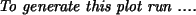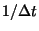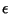Next: 5.1.3 Display and Initial Up: 5.1 N-body experiments Previous: 5.1.1 Setting it up   Contents   Index

## 5.1.2 Integration using hackcode1

We then run the collision for 20 time units, with the standard N-body integrator based on the Barnes & Hut "hierarchical tree" algorithm5.4:

    7% hackcode1 in=i001.dat out=r001.dat tstop=20 freqout=2 \
freq=40 eps=0.05 tol=0.7 options=mass,phase,phi > r001.log


The integration frequency relates to the integration timestep as freq =, the softening length eps =, and opening angle or tolerance tol =. A major output of masses, positions and potentials of all particles is done every 1/freqout = 0.5 time units, which corresponds to about 1/5 of a crossing time. The standard output of the calculation is diverted to a file r001.log for convenience. This is an (ASCII) listing, containing useful statistics of the run, such as the efficiency of the force calculation, conserved quantities etc. Some of this information is also stored in diagnostic sets in the structured binary output file r001.dat.

As an exercise, compare the output of the following two commands:

    8% more r001.log
9% tsf r001.dat | more


(c) Peter Teuben